Class, Below is a YOUTUBE video that explains Ventricular Volumes and Ejection Fraction (EF) calculations as well as some descriptive graphics.

Ventricular Volumes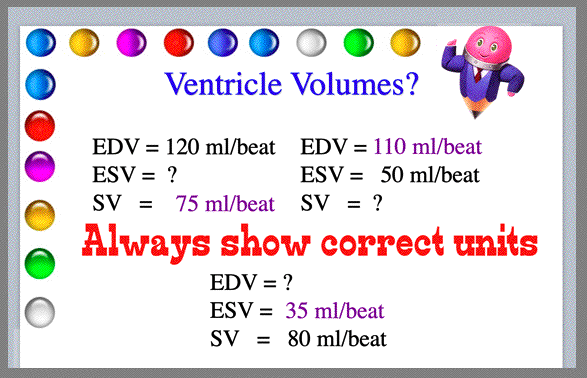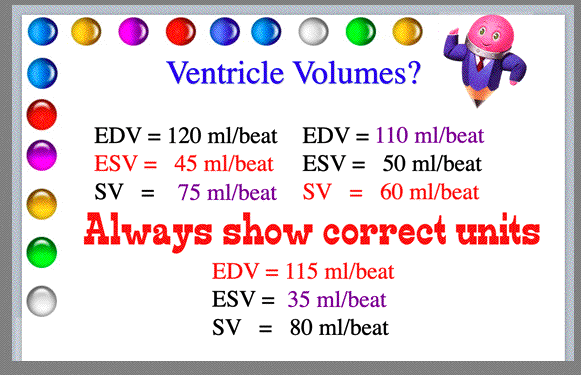With Venticlar volumes, if you have two volumes you can always solve for one unknown volume. This is basic algebra: SV = EDV - ESV

Stroke volume: Blood ejected from heart (left ventricle) per beat (the average is 60-80 ml/beat)

EDV: End-diastolic volume (ventricle is filled)

ESV: End-systolic volume (blood left in ventricle after ejectionNow, see if you can solve a few word questions with ventricular volumes.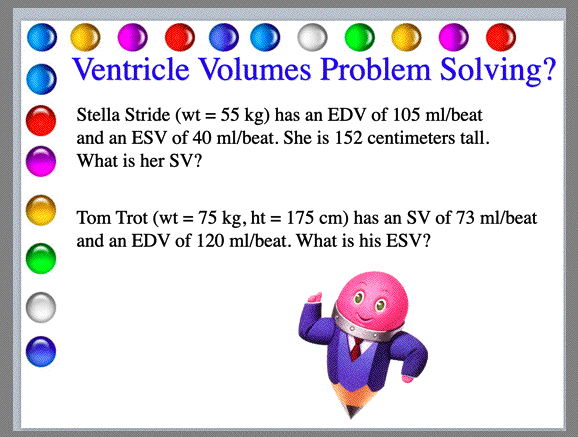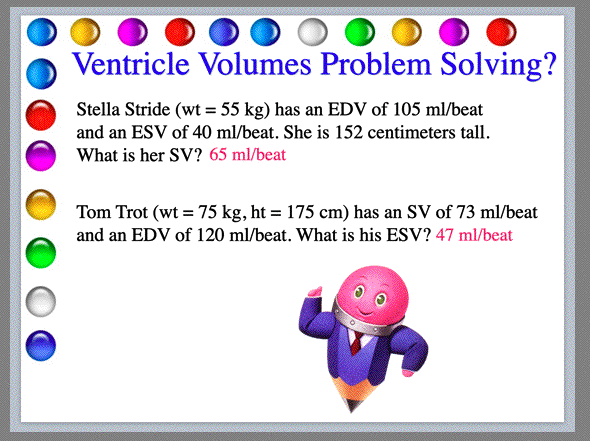Ejection Fraction Calculations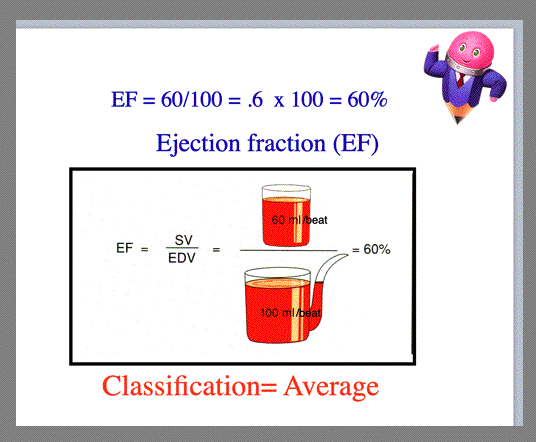Ejection fraction is solved by dividing Stroke Volume (SV) by End Diastolic Volume (EDV). Then, you multiply your calculation by 100 to get the percent. Ejection faction is presented in a percent.

An average ejection fraction classification is 60%

If the ejection fraction is higher than 60% then the classification is Above average

If the ejection fraction is less than 60% then the classification is Below average.
Now, try working a word problem with ejection fraction.Top of Page | Research Interests | Vita | Articles | New Projects | Miscellaneous | UNM | Home